# R: Matrix Operations

Imagine the following problem: You have a business assembling cabinets. You have 10 employees and you want to see who is the most efficient. You track the number of cabinets assembled by each employee over 10 days. You also track the hours they worked each day in a separate table.

Let’s create our tables –

Number of Cabinets

Create a vector of 100 random data points from 50 to 100.

```# created random set of 100 numbers between 50 and 100
x <- sample(50:100,100, T)
x```

sample(range,number of data, allow repeat of data (T,F))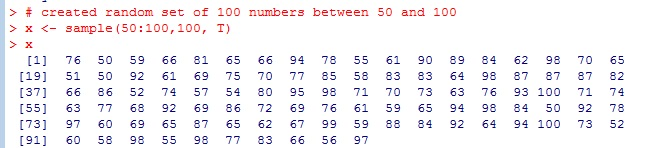Convert to a matrix

```# convert to a 10x10 matrix
A <- matrix(x,10,10)
A```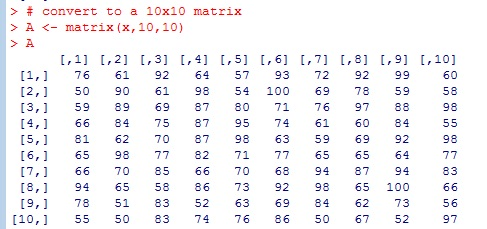Name Columns and Rows

```#name rows and columns
colnames(A) <- c("D1","D2","D3","D4","D5","D6","D7","D8","D9","D10")
row.names(A) <- c("Bob","Steve","Gary","Sara","Tony","Stacey","Kerri","Debbie","George","Manny")
A```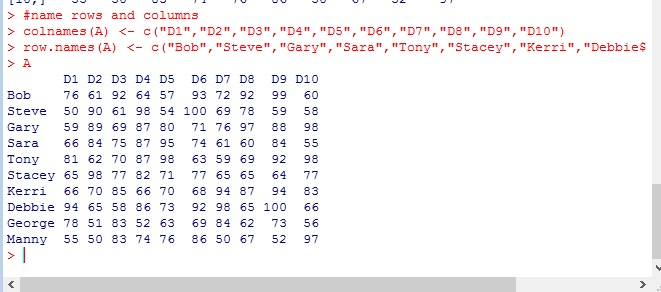Hours Worked

Let’s do the same thing, but now build our hours table.

```# created random set of 100 numbers between 1 and 9
y <- sample(1:9,100, T)
y```Convert to 10×10 matrix form

```# convert to a 10x10 matrix
B <- matrix(y,10,10)
B```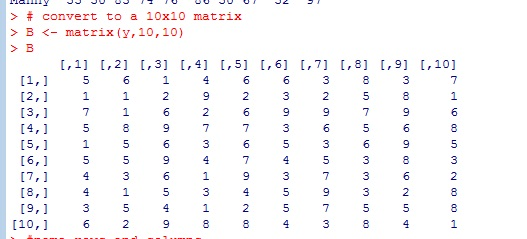Name our columns and rows

```#name rows and columns
colnames(B) <- c("D1","D2","D3","D4","D5","D6","D7","D8","D9","D10")
row.names(B) <- c("Bob","Steve","Gary","Sara","Tony","Stacey","Kerri","Debbie","George","Manny")
B```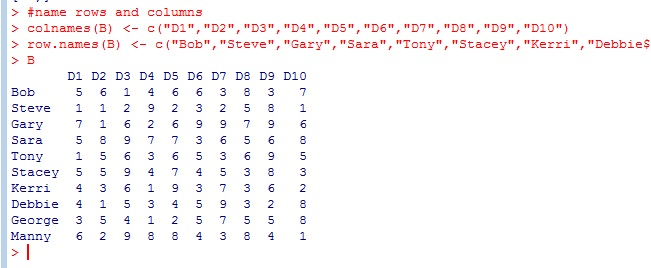## Matrix Operations

Now we will see the magic of R. This programming language was built for Statisticians. So it has some great matrix operations that make linear algebra operations a breeze. In fact, if you ever took linear algebra, you will wish you had known about R back then.

Now if I want to know how many cabinets each person made per hour worked on any given day, I would need to divide each elements of matrix A by its corresponding element in matrix B.

Taking what you know about For loops, think for a minute what the loop would look like to complete this task. Messy huh?

Well in R, we don’t have to worry it. Because in a R, A/B does just what we are asking for.

```#matrix division
C = A/B
C```The results are bit much, let’s round it to 1 decimal place

```#round Matrix
round(C, digits =1)```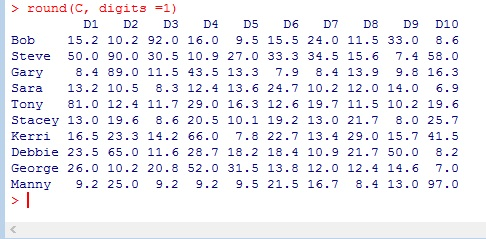Okay, now let’s find the most and least productive values

```#most and least
max(C)
min(C)```

So we have one person who made 97 cabinets in an hour and another who made 6.875Who are they? And what day was it?

```#who are they?
which(C==max(C), arr.ind=T)
which(C==min(C), arr.ind=T)```

Manny is our hero on day 10, and Sara must have been hung over on day 10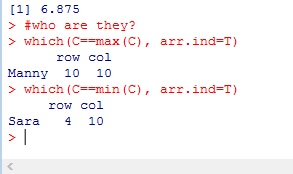I know, I know, if you are here, then you like me are a stat geek. So let’s run the mean and see who is the overall Rockstar.

```#get the means
colMeans(C)
rowMeans(C)```

I have underlined highest and lowest, both day and employee. Looks like Sara is a real slacker.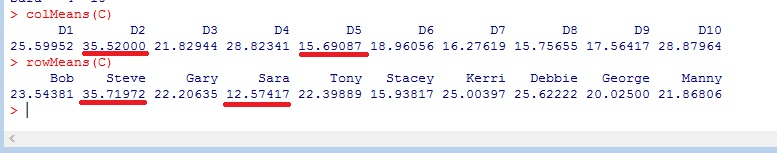Now before you start sending hate mail and calling me sexist, remember these numbers were randomly generated. If I ran my code again, I would get completely different answers. Just as if you follow along, your answers will not match up with mine.

## The Code

```# created random set of 100 numbers between 50 and 100
x <- sample(50:100,100, T)
x
# convert to a 10x10 matrix
A <- matrix(x,10,10)
A
#name rows and columns
colnames(A) <- c("D1","D2","D3","D4","D5","D6","D7","D8","D9","D10")
row.names(A) <- c("Bob","Steve","Gary","Sara","Tony","Stacey","Kerri","Debbie","George","Manny")
A

# created random set of 100 numbers between 1 and 9
y <- sample(1:9,100, T)
y
# convert to a 10x10 matrix
B <- matrix(y,10,10)
B
#name rows and columns
colnames(B) <- c("D1","D2","D3","D4","D5","D6","D7","D8","D9","D10")
row.names(B) <- c("Bob","Steve","Gary","Sara","Tony","Stacey","Kerri","Debbie","George","Manny")
B

#matrix division
C = A/B
C

#round Matrix
round(C, digits =1)

#most and least productive values
max(C)
min(C)

#who are they?
which(C==max(C), arr.ind=T)
which(C==min(C), arr.ind=T)

#get the means
colMeans(C)
rowMeans(C)```